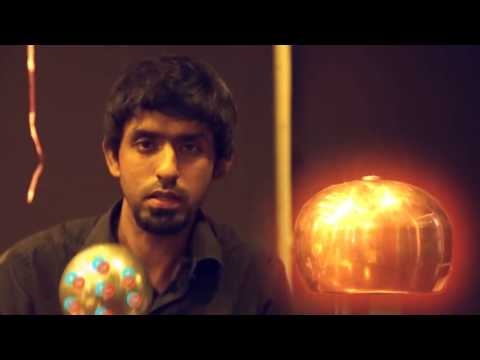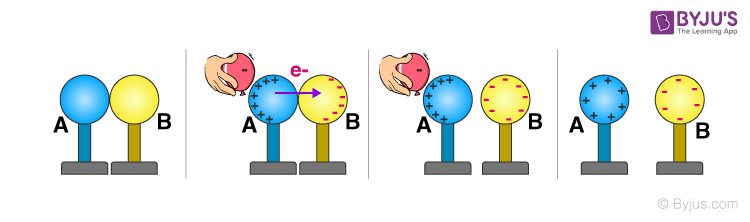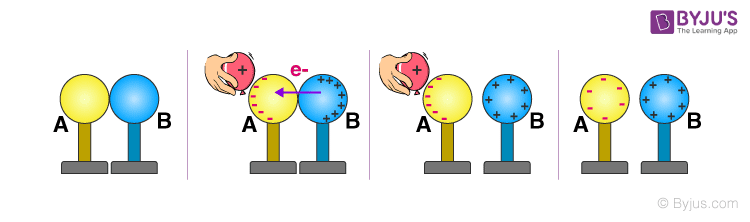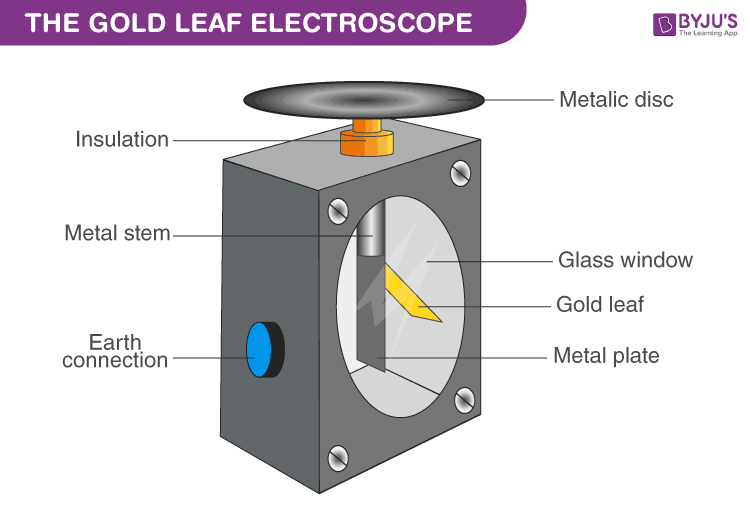# Charging by Induction - Negative and Positive Charges

Most objects are electrically neutral, which means that they have an equal number of positive and negative charges. In order to charge an object, one has to alter the charge balance of positive and negative charges. There are three ways to do it: friction, conduction and induction.

### Charging by Friction:

The charging by friction process involves rubbing of one particle on another resulting in electrons moving from one surface to another. This method is useful for charging insulators.

### Charging by Conduction:

The charging by conduction process involves touching of a charged particle to a conductive material. This way, the charges are transferred from the charged material to the conductor. This method is useful for charging conductors.

### Charging By Induction:

Lastly, the induction charging is a charging method that charges an object without actually touching the object to any another charged object. The charging by induction process is where the charged particle is held near an uncharged conductive material that is grounded on a neutrally charged material. The charge flows between two objects and the uncharged conductive material develop a charge with opposite polarity.

These were the different methods of charge transfer. Here, in the article let us learn more about the method of charging by induction.

Learn the basics of Charging by Induction through the visualization technique explained in this video.## Charging by induction using a negatively charged object

In this section, we shall learn about the transfer of charge by induction using a negatively charged object. Let us consider two metal spheres A and B touching each other, as shown in the figure. Let us take a negatively charged rubber balloon. If we bring the charged balloon near the spheres, electrons within the two-sphere system will be induced to move away from the balloon due to the repulsion between the electrons of the balloon and the spheres. Subsequently, the electrons from sphere A get transferred to sphere B.The migration of electrons causes the sphere A to become positively charged and the sphere B to be negatively charged. The overall two-sphere system is hence electrically neutral. The spheres are then separated using an insulating cover such as gloves or a stand as shown in the figure (avoiding direct contact with the metal). When we remove the balloon, the charge gets redistributed, spreading throughout the spheres, as shown in the figure.When a negatively charged balloon is brought near the sphere system, the electrons in the sphere will be forced to move away due to repulsion. The migration of electrons causes sphere A to become completely positive and sphere B to become negative.

## Charging by induction using a positively charged object

In this section, we shall learn about the transfer of charge through the process of induction using a positively charged object. Taking two spheres A and B, touching each other, as shown in the figure, if we bring a positively charged balloon near sphere A, the electrons from sphere B migrate towards sphere A due to the attraction between opposite charges, thus leaving the sphere B deficit of electrons. As a result, the sphere A is negatively charged and the sphere B is positively charged. The spheres are then separated using an insulating cover, a stand or gloves. When the balloon is removed, the charges in sphere A and B redistribute, spreading out evenly.When a positively charged balloon is brought near the sphere system, the electrons from sphere B will migrate towards sphere A due to the force of attraction. Now, sphere A is negatively charged and the sphere B is positively charged.

## Charging of Electroscope by InductionAn electroscope is an instrument that is used to detect the presence and magnitude of electric charges on a body. An electroscope is commonly used by physics teachers to demonstrate the electrostatic principles of charging and charge interactions.

The demonstration of the induction process of charging is commonly performed with an electroscope. In this demonstration, a charged particle is brought close to but not touching the electroscope. The electrons in the electroscope are induced to move due to the presence of the charged particle above the plate of the electroscope. With the charged particle still held above the plate, the electroscope is touched. At this point, electrons will flow between the electroscope and the ground, giving the electroscope an overall charge. The needle of the electroscope deflects indicating an overall charge when the charged particle is pulled away from it. From this demonstration, the following fundamental principles can be summarised:

• The object being charged by induction never touches the charged particle.
• The charged object does not transfer electrons to or receive electrons from the object being charged.
• The object being charged is touched by a ground; electrons are transferred between the ground and the object being charged (either into the object or out of it).
• The charged object serves to polarize the object being charged.
• The object being charged ultimately receives a charge that is opposite that of the charged object that is used to polarize it.

The application of inductive charging can be divided into high power and low power categories. The low power applications generally include handheld devices, phones, computer and other devices which charge at power levels below 100 watts. High power inductive charging applications include charging of batteries of power levels above 1 kilowatt. The most prominent application is the charging of electric vehicles where an automated and wireless inductive charging is provided as an alternative to plug-in charging.

## Review Questions

• List the various differences between electrostatic and electromagnetic induction.
 Electromagnetic Induction Electrostatic Induction The generation of emf in a conductor due to the rate of change of current in a nearby conductor without any electrical connection. The accumulation or redistribution of electric charges in a body due to nearby charged body without any physical contact. It works for long distances It works for short distances It is due to the rate of change of flow of charges It is due to static charges The effect is maximum in conductors The effect is maximum in insulators Electric fields of the charges are the reason Magnetic fields due to moving charges are the reason
• Which instrument is used to detect the nature of the charge?

Electroscope is used to detect the nature of the charge.

• How is charging by induction method used in real life?

The charging by induction method is used in charging of microphones, smartphones, etc. As induction method is the transfer of charge from one object to another so by placing the phone on dock charge transfer from dock to phone.